## 3D Shapes

A 3D shape is described by its edges, faces, and vertices (vertex is the singular form of vertices).

This video and images below explain the faces, vertices and edges of common three-dimensional shapes.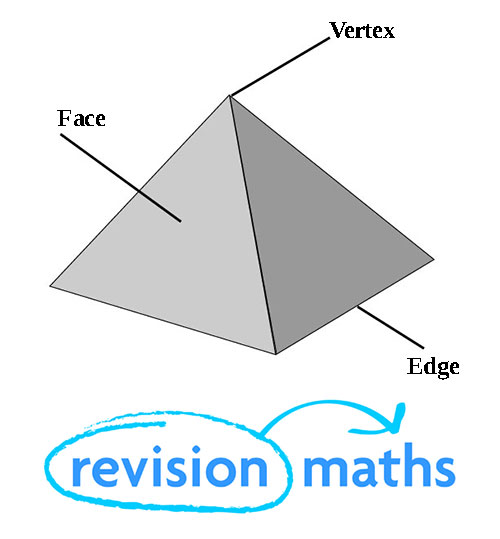A face is a 2D shape that makes up one surface of a 3D shape, an edge is where two faces meet and a vertex is the point or corner of a geometric shape.

To work out the area of a face of a 3D shape, you use square units such as cm2 as the face of a 3D shape is a 2D shape in its own right (a pyramid’s face will form a triangle, or its base a square). You can learn how to work out area of a shape here.

To work out the volume of the whole 3D shape you used cubic units such as cm3. To learn how to work out the volume of 3D shapes click here.

Properties of 3D Shapes – Prisms

Cube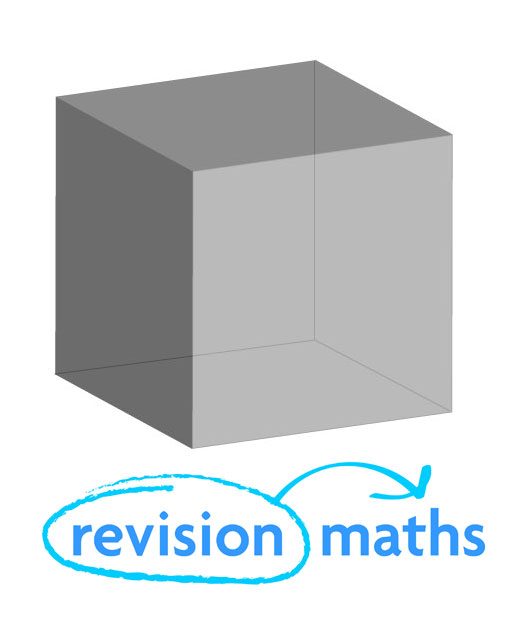Number of Edges: 12

Number of Faces: 6

Number of Vertices: 8

Cuboid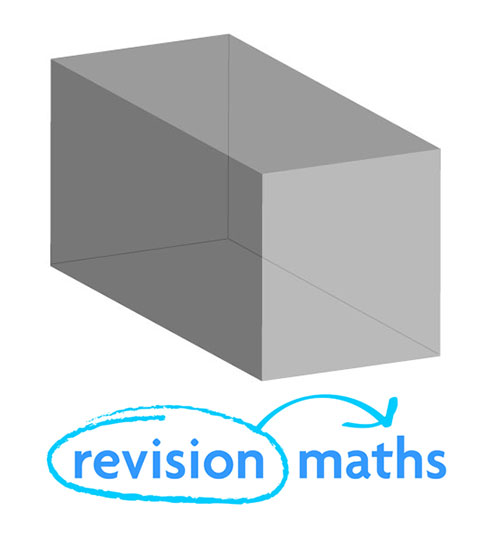Number of Edges: 12

Number of Faces: 6

Number of Vertices: 8

Cylinder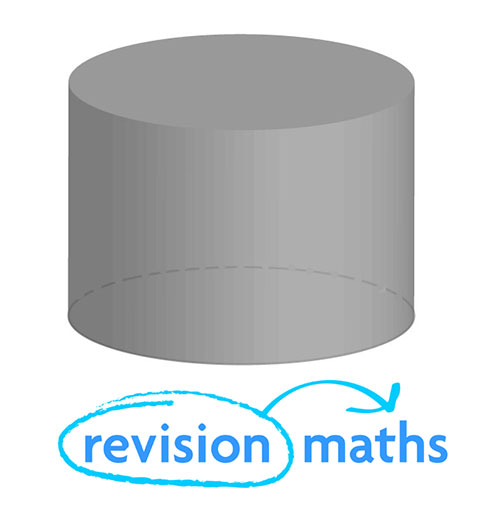Number of Edges: 2

Number of Faces: 3

Number of Vertices: 0

Triangular Prism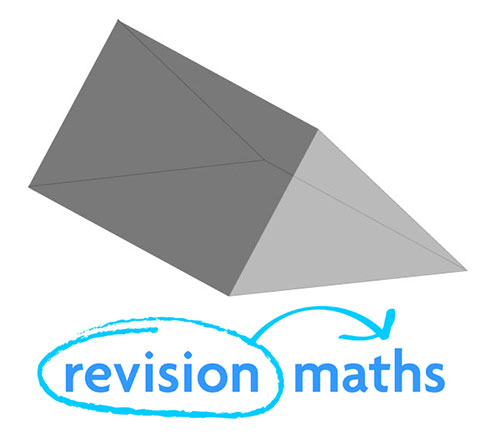Number of Edges: 9

Number of Faces: 5

Number of Vertices: 6

Octagonal Prism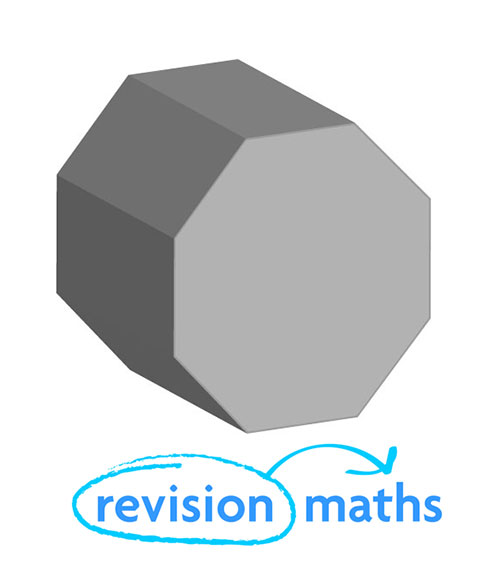Number of Edges: 24

Number of Faces: 10

Number of Vertices: 16

Properties of 3D Shapes – Pyramids

Tetrahedron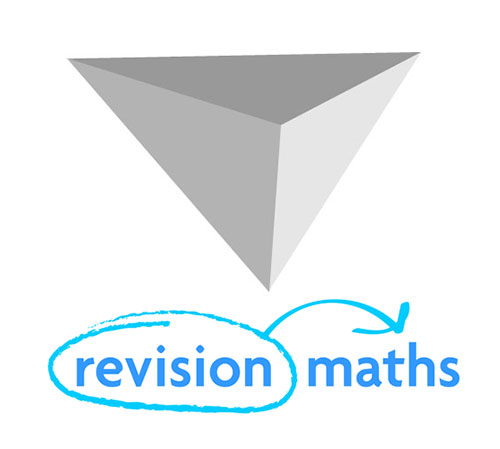Number of Edges: 6

Number of Faces: 4

Number of Vertices: 4

Square based Pyramid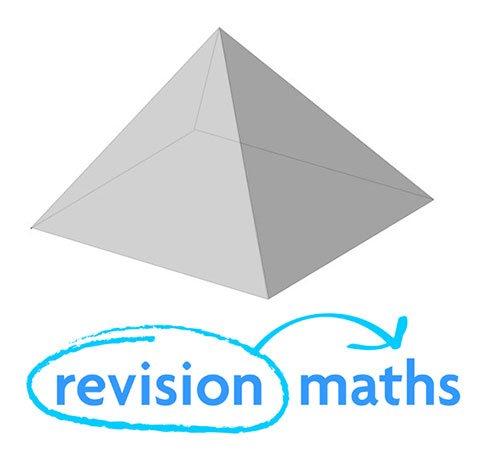Number of Edges: 8

Number of Faces: 5

Number of Vertices: 5

Hexagonal based Pyramid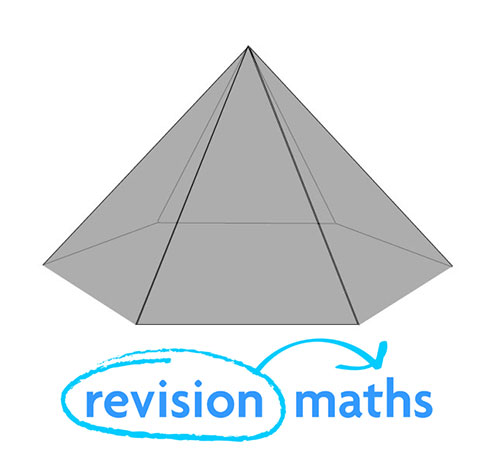Number of Edges: 12

Number of Faces: 7

Number of Vertices: 7

Properties of 3D Shapes – Curved Faces

Cone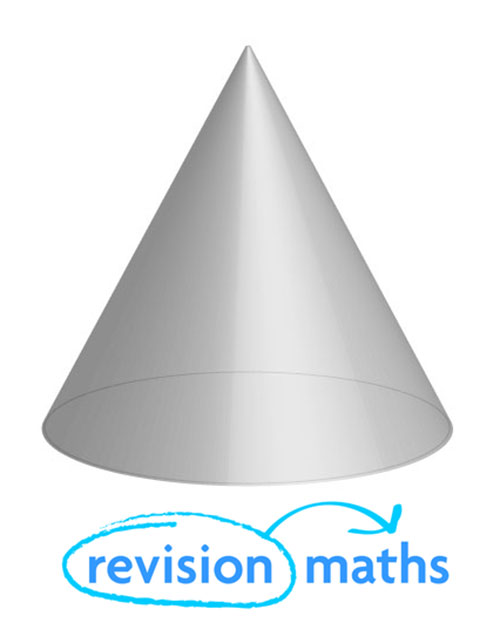Number of Edges: 1

Number of Faces: 2

Number of Vertices: 1

Frustum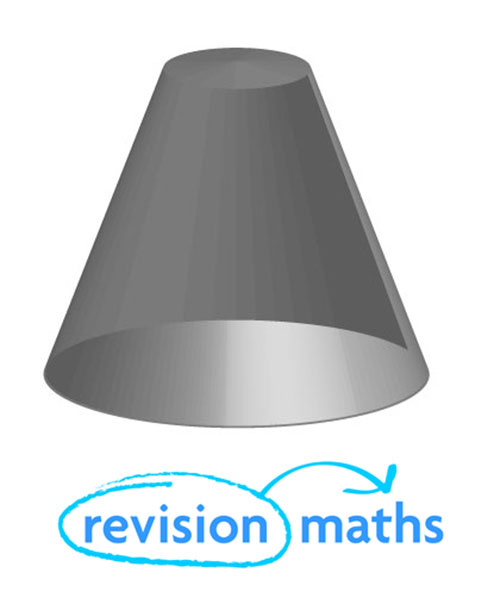Number of Edges: 2

Number of Faces: 3

Number of Vertices: 0

Sphere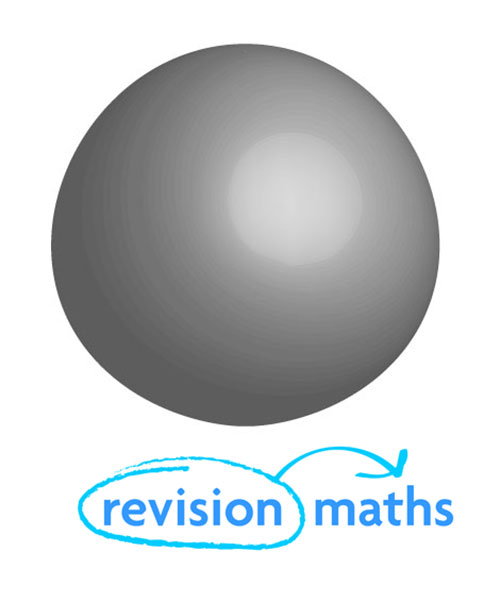Number of Edges: 0

Number of Faces: 1

Number of Vertices: 0